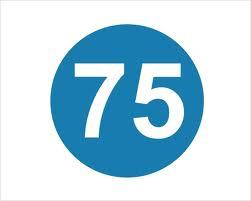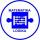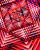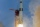# Sequence 3

Write the first 5 members of an arithmetic sequence: a4=-35, a11=-105.

a1 =  -5
a2 =  -15
a3 =  -25
a4 =  -35
a5 =  -45

### Step-by-step explanation:

${a}_{2}={a}_{1}-10=-15$
${a}_{3}={a}_{2}-10=-25$
${a}_{4}={a}_{3}-10=-35$
${a}_{5}={a}_{4}-10=-45$Did you find an error or inaccuracy? Feel free to write us. Thank you!## Related math problems and questions:

• Sequence 2Write the first 5 members of an arithmetic sequence a11=-14, d=-1
• AP - simpleFind the first ten members of the sequence if a11 = 132, d = 3.
• SequenceWrite the first 6 members of this sequence: a1 = 5 a2 = 7 an+2 = an+1 +2 an
• SequenceWrite the first 7 members of an arithmetic sequence: a1=-3, d=6.
• Sequence - 5 membersWrite first five members of the sequence ?
• SequenceBetween numbers 1 and 53 insert n members of the arithmetic sequence that its sum is 702.
• Five membersWrite first 5 members geometric sequence and determine whether it is increasing or decreasing: a1 = 3 q = -2
• AS sequenceIn an arithmetic sequence is given the difference d = -3 and a71 = 455. a) Determine the value of a62 b) Determine the sum of 71 members.
• Finite arithmetic sequenceHow many numbers should be inserted between the numbers 1 and 25 so that all numbers create a finite arithmetic sequence and that the sum of all members of this group is 117?
• AS - sequenceWhat are the first ten members of the sequence if a11=22, d=2.
• Sequences AP + GPThe three numbers that make up the arithmetic sequence have the sum of 30. If we subtract from the first 5, from the second 4 and keep the third, we get the geometric sequence. Find AP and GP members.
• Geometric sequence 3In geometric sequence is a8 = 312500; a11= 39062500; sn=1953124. Calculate the first item a1, quotient q, and n - number of members by their sum s_n.
• GP - sequenceThe first three terms of a geometric sequence are as follows 10, 30. 90, find the next two terms of this sequence.
• GP membersThe geometric sequence has 10 members. The last two members are 2 and -1. Which member is -1/16?
• In theIn the arithmetic sequence a1 = 4.8, d = 0.4. How many consecutive members, starting with the first, need to be added so that the sum is greater than 170?
• Geometric sequence 4It is given geometric sequence a3 = 7 and a12 = 3. Calculate s23 (= sum of the first 23 members of the sequence).
• The sumThe sum of the first 10 members of the arithmetic sequence is 120. What will be the sum if the difference is reduced by 2?